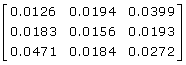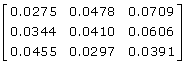# Table 4 Multiplicative model (MULT).

Low risk High risk
a1 = 2· a0; a2 = 4· a0 a1 = 5· a0; a2 = 10· a0
b1 = 5· b0; b2 = 10· b0 b1 = 5· b0; b2 = 10· b0
Theoretical penetrance matrixNeural network
Mean absolute difference ESum 0.2428 0.2178
Logistic regression
Mean absolute difference ESum 0.3965 0.4887
Logistic regression (design variables)
Mean absolute difference ESum 0.1637 0.1833
1. Mean absolute differences between theoretical and estimated penetrance matrices from 100 replications in the low and high risk scenario.